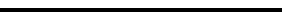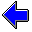Java Security Settings:
This web page employs Java, which requires specific security settings for correct operation.
If the applets on this page do not run correctly, consult the Virtual Chemistry Experiments FAQ
or the Physlet Physics web site for establishing the correct security settings.

# Gas Laws

## Pressure

### Concepts

Pressure ( P ) is the ratio of the force ( F ) exerted upon a surface to the surface area ( A ).

P = F / A
 A manometer is an device employed to measure pressure. There are a variety of manometer designs. A simple, common design is to seal a length of glass tubing and bend the glass tube into a U-shape. The glass tube is then filled with a liquid, typically mercury, so that all trapped air is removed from the sealed end of the tube. The glass tube is then positioned with the curved region at the bottom. The mercury settles to the bottom (see the picture at the left). After the mercury settles to the bottom of the manometer, a vacuum is produced in the sealed tube (the left tube in the picture). The open tube is connected to the system whose pressure is being measured. In the sealed tube, there is no gas to exert a force on the mercury (except for some mercury vapor). In the tube connected to the system, the gas in the system exerts a force on the mercury. The net result is that the column of mercury in the left (sealed) tube is higher than that in the right (unsealed) tube. The difference in the heights of the columns of mercury is a measure of the pressure of gas in the system. In the example at the left, the top of the left column of mercury corresponds to 875 mm on the scale. The top of the right column of mercury corresponds to 115 mm. The difference in heights is 875 mm - 115 mm = 760. mm, which indicates that the pressure is 760. mm Hg or 760. torr.

This method for measuring pressure led to the use of millimeters of mercury (mm Hg) as a unit of pressure. Today 1 mm Hg is called 1 torr. A pressure of 1 torr or 1 mm Hg is literally the pressure that produces a 1 mm difference in the heights of the two columns of mercury in a manometer.

To understand how the height of a column of mercury can be used as a unit of pressure and how the unit of torr is related to the SI unit of pascal (1 Pa = 1 N/m2), consider the following mathematical analysis of the behavior of the manometer.

The force exerted by the column of mercury in a tube arises from the gravitational acceleration of the column of mercury. Newton's Second Law provides an expression for this force:

F = m g

In this equation, m is the mass of mercury in the column and g = 9.80665 m/sec2 is the gravitational acceleration. This force is distributed over the cross-sectional area of the column ( A ). The pressure resulting from the column of mercury is thus

 P = m gA

The mass of mercury is given by the product of the density of mercury ( dHg ) and the volume of mercury ( V ). For a cylindrical column of mercury, the volume of mercury is the product of the cross-sectional area and the height of the column ( h ). These relationships product the following equation.

 P = m gA = dHg V gA = dHg A h gA = dHg h g

This equation clearly shows that the height of a column of mercury is directly proportional to the pressure exerted by that column of mercury. The difference in heights of the two columns of mercury in a manometer can thus be used to measure the difference in pressures between the two sides of the manometer.

The relation between torr and Pa is also clearly evident. Using dHg = 13.5951 g cm-3, one finds that 1 torr = 133 Pa or 1 atm = 760 torr = 101 kPa.

.

## Part 1: Reading a Manometer

 Objective Use a manometer to measure pressure Carefully read the heights of the two columns of mercury in the manometer at the right. Note that the scale is in units of millimeters. Use the two heights to determine the pressure of the system. Calculate the pressure in torr, atm, kPa, and psi. After determining the pressure, select the "Show Pressure" button to show the actual pressure. Did you measure the pressure correctly? Select the "New Pressure" button to obtain a new pressure and repeat the exercise. Practice making measurements and converting between units until you can consistently obtain accurate values. Bear in mind that there is a limit to the precision with which you can read the scale. Consequently there will some uncertainty in your reading. Your value should be close to the correct value, but there will be some error.

## Part 2: Using a Manometer containing Water

 Objective Use a manometer that contains water to measure pressure This part of the experiment is identical to Part 1 with the exception that the manometer contains water rather than mercury. The difference in the heights of water in the two columns provides the pressure in units of mm H2O. Carefully examine the mathematical analysis presented in the Concepts section to devise a conversion factor to transform the pressure in units of mm H2O to units of mm Hg or torr. (Assume the density of water is 1.00 g cm-3.) Carefully read the heights of the two columns of water in the manometer at the right. Note that the scale is in units of millimeters. Use the two heights to determine the pressure of the system. After trying several different pressures, do you notice a significant difference between the results in Part 1 and those in this exercise? What practical difference results from using water rather than mercury?

## Part 3: Effect of Vapor Pressure

 Objective Measure pressure using a water-filled manometer and taking the vapor pressure of water into account. As you hopefully observed in Part 2, one of the notable differences between mercury and water is density. Because mercury is much denser than water, a column of mercury weighs more. Another important difference is the vapor pressure. Molecules from a liquid can escape into the gas phase, and those gas-phase molecules exert a pressure called the vapor pressure. The existence of a vapor pressure means that the "void" space above the liquid in the closed end of the manometer is not really a vacuum. This region actually contains a small amount of vapor, which itself exerts a pressure on the liquid in the closed tube. A major advantage to using mercury in a manometer is the low vapor pressure of mercury, which is 0.0017 torr at 25 oC. This small vapor pressure can be ignored for all but very low pressure measurements. The vapor pressure of water is 23.8 torr at 25 oC, a value which is obviously significant considering your measurements in Part 2. Repeat the measurements from Part 2 taking into account the vapor pressure of water. (Note that the gas in the open-ended tube on the right also contains water vapor. In this case you are determining the total pressure of the gas mixture.)

Boyle's LawGas Laws Home PageVirtual Chemistry Home Page

Pressure.html version 2.1